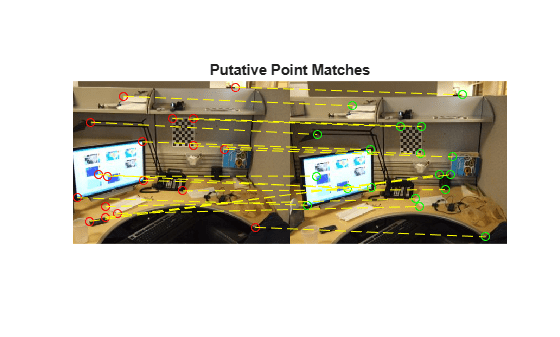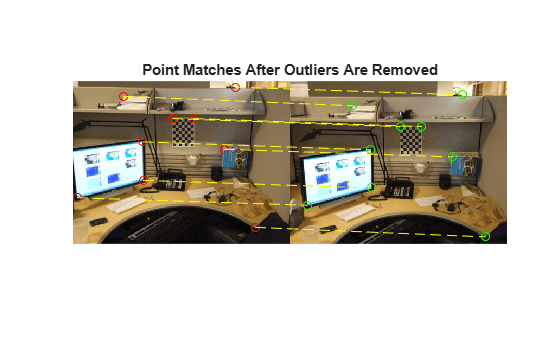# estimateFundamentalMatrix

Estimate fundamental matrix from corresponding points in stereo images

## Syntax

``estimateFundamentalMatrix``
``F = estimateFundamentalMatrix(matchedPoints1,matchedPoints2)``
``````[F,inliersIndex] = estimateFundamentalMatrix(matchedPoints1,matchedPoints2)``````
``````[F,inliersIndex,status] = estimateFundamentalMatrix(matchedPoints1,matchedPoints2)``````
``````[F,inliersIndex,status] = estimateFundamentalMatrix(matchedPoints1,matchedPoints2,Name,Value)``````

## Description

example

````estimateFundamentalMatrix` estimates the fundamental matrix from corresponding points in stereo images. This function can be configured to use all corresponding points or to exclude outliers. You can exclude outliers by using a robust estimation technique such as random-sample consensus (RANSAC). When you use robust estimation, results may not be identical between runs because of the randomized nature of the algorithm.```

example

````F = estimateFundamentalMatrix(matchedPoints1,matchedPoints2)` returns the 3-by-3 fundamental matrix, `F`, using the least median of squares (LMedS) method. The input points can be M-by-2 matrices of M number of [x y] coordinates, or `KAZEPoints`, `SURFPoints`, `MSERRegions`, `ORBPoints`, or `cornerPoints` object.```
``````[F,inliersIndex] = estimateFundamentalMatrix(matchedPoints1,matchedPoints2)``` additionally returns logical indices, `inliersIndex`, for the inliers used to compute the fundamental matrix. The `inliersIndex` output is an M-by-1 vector. The function sets the elements of the vector to `true` when the corresponding point was used to compute the fundamental matrix. The elements are set to `false` if they are not used.```
``````[F,inliersIndex,status] = estimateFundamentalMatrix(matchedPoints1,matchedPoints2)``` additionally returns a status code.```
``````[F,inliersIndex,status] = estimateFundamentalMatrix(matchedPoints1,matchedPoints2,Name,Value)``` uses additional options specified by one or more Name,Value pair arguments.```

## Examples

collapse all

The RANSAC method requires that the input points are already putatively matched. We can, for example, use the `matchFeatures` function for this. Using the RANSAC algorithm eliminates any outliers which may still be contained within putatively matched points.

`load stereoPointPairs`

Estimate the fundamental matrix.

```fRANSAC = estimateFundamentalMatrix(matchedPoints1,... matchedPoints2,'Method','RANSAC',... 'NumTrials',2000,'DistanceThreshold',1e-4)```
```fRANSAC = 3×3 0.0000 -0.0004 0.0348 0.0004 0.0000 -0.0937 -0.0426 0.0993 0.9892 ```

```load stereoPointPairs [fLMedS, inliers] = estimateFundamentalMatrix(matchedPoints1,matchedPoints2,'NumTrials',2000)```
```fLMedS = 3×3 0.0000 -0.0004 0.0349 0.0004 0.0000 -0.0938 -0.0426 0.0994 0.9892 ```
```inliers = 18x1 logical array 1 1 1 1 1 1 0 1 0 0 ⋮ ```

```I1 = imread('viprectification_deskLeft.png'); I2 = imread('viprectification_deskRight.png');```

Show the putatively matched points.

```figure; showMatchedFeatures(I1,I2,matchedPoints1,matchedPoints2,'montage','PlotOptions',{'ro','go','y--'}); title('Putative point matches');```Show the inlier points.

```figure; showMatchedFeatures(I1, I2, matchedPoints1(inliers,:),matchedPoints2(inliers,:),'montage','PlotOptions',{'ro','go','y--'}); title('Point matches after outliers were removed');````load stereoPointPairs`

Compute the fundamental matrix for input points which do not contain any outliers.

```inlierPts1 = matchedPoints1(knownInliers,:); inlierPts2 = matchedPoints2(knownInliers,:); fNorm8Point = estimateFundamentalMatrix(inlierPts1,inlierPts2,'Method','Norm8Point')```
```fNorm8Point = 3×3 0.0000 -0.0004 0.0348 0.0004 0.0000 -0.0937 -0.0426 0.0993 0.9892 ```

## Input Arguments

collapse all

Coordinates of corresponding points in image one, specified as an M-by-2 matrix of M number of [x y] coordinates, or as a `KAZEPoints`, `SURFPoints`, `MSERRegions`, `ORBPoints`, or `cornerPoints` object. The `matchedPoints1` input must contain points which do not lie on a single planar surface, (e.g., a wall, table, or book) and are putatively matched by using a function such as `matchFeatures`.

Coordinates of corresponding points in image two, specified as an M-by-2 matrix of M number of [x y] coordinates, or as a `KAZEPoints`, `SURFPoints`, `MSERRegions`, `ORBPoints`, or `cornerPoints` object. The `matchedPoints2` input must contain points which do not lie on a single planar surface, (e.g., a wall, table, or book) and are putatively matched by using a function such as `matchFeatures`

### Name-Value Pair Arguments

Specify optional comma-separated pairs of `Name,Value` arguments. `Name` is the argument name and `Value` is the corresponding value. `Name` must appear inside quotes. You can specify several name and value pair arguments in any order as `Name1,Value1,...,NameN,ValueN`.

Example: `'Method'`, '`RANSAC`' specifies RANSAC as the method to compute the fundamental matrix.

Method used to compute the fundamental matrix, specified as the comma-separated pair consisting of '`Method`' and one of the following:

 `Norm8Point` Normalized eight-point algorithm. To produce reliable results, the inputs, `matchedPoints1` and `matchedPoints2` must match precisely. `LMedS` Least Median of Squares. Select this method if you know that at least 50% of the points in `matchedPoints1` and `matchedPoints2` are inliers. `RANSAC` RANdom SAmple Consensus. Select this method if you would like to set the distance threshold for the inliers. `MSAC` M-estimator SAmple Consensus. Select the M-estimator SAmple Consensus method if you would like to set the distance threshold for the inliers. Generally, the MSAC method converges more quickly than the RANSAC method. `LTS` Least Trimmed Squares. Select the Least Trimmed Squares method if you know a minimum percentage of inliers in `matchedPoints1` and `matchedPoints2`. Generally, the LTS method converges more quickly than the LMedS method.

To produce reliable results using the `Norm8Point` algorithm, the inputs, `matchedPoints1` and `matchedPoints2`, must match precisely. The other methods can tolerate outliers and therefore only require putatively matched input points. You can obtain putatively matched points by using the `matchFeatures` function.

Fundamental matrix class, specified as the comma-separated pair consisting of '`OutputClass`' and either `'double'` or `'single'`. This specifies the class for the fundamental matrix and the function's internal computations.

Number of random trials for finding the outliers, specified as the comma-separated pair consisting of '`NumTrials`' and an integer value. This parameter applies when you set the `Method` parameter to `LMedS`, `RANSAC`, `MSAC`, or `LTS`.

When you set the `Method` parameter to either `LMedS` or `LTS`, the function uses the actual number of trials as the parameter value.

When you set the `Method` parameter to either `RANSAC` or `MSAC`, the function uses the maximum number of trials as the parameter value. The actual number of trials depends on `matchedPoints1`, `matchedPoints2`, and the value of the `Confidence` parameter.

Select the number of random trials to optimize speed and accuracy.

Algebraic or Sampson distance type, specified as the comma-separated pair consisting of '`DistanceType`' and either the `Algebraic` or `Sampson`. The distance type determines whether a pair of points is an inlier or outlier. This parameter applies when you set the `Method` parameter to `LMedS`, `RANSAC`, `MSAC`, or `LTS`.

Note

For faster computations, set this parameter to `Algebraic`. For a geometric distance, set this parameter to `Sampson`.

Data Types: `char`

Distance threshold for finding outliers, specified as the comma-separated pair consisting of '`DistanceThreshold`' and a positive value. This parameter applies when you set the `Method` parameter to `RANSAC` or `MSAC`.

Desired confidence for finding maximum number of inliers, specified as the comma-separated pair consisting of '`Confidence`' and a percentage scalar value in the range (0 100). This parameter applies when you set the `Method` parameter to `RANSAC` or `MSAC`.

Minimum percentage of inliers in input points, specified as the comma-separated pair consisting of '`InlierPercentage`' and percentage scalar value in the range (0 100). Specify the minimum percentage of inliers in `matchedPoints1` and `matchedPoints2`. This parameter applies when you set the `Method` parameter to `LTS`.

Report runtime error, specified as the comma-separated pair consisting of '`ReportRuntimeError`' and a logical value. Set this parameter to `true` to report run-time errors when the function cannot compute the fundamental matrix from `matchedPoints1` and `matchedPoints2`. When you set this parameter to `false`, you can check the `status` output to verify validity of the fundamental matrix.

## Output Arguments

collapse all

Fundamental matrix, returns as a 3-by-3 matrix that is computed from the points in the inputs `matchedPoints1` and `matchedPoints2`.

`$\left[\begin{array}{cc}{P}_{2}& 1\end{array}\right]*FundamentalMatrix*{\left[\begin{array}{cc}{P}_{1}& 1\end{array}\right]}^{\text{'}}=0$`

P1, the point in `matchedPoints1` of image 1 in pixels, corresponds to the point, P2, the point in `matchedPoints2` in image 2.

In computer vision, the fundamental matrix is a 3-by-3 matrix which relates corresponding points in stereo images. When two cameras view a 3-D scene from two distinct positions, there are a number of geometric relations between the 3-D points and their projections onto the 2-D images that lead to constraints between the image points. Two images of the same scene are related by epipolar geometry.

Inliers index, returned as an M-by-1 logical index vector. An element set to `true` means that the corresponding indexed matched points in `matchedPoints1` and `matchedPoints2` were used to compute the fundamental matrix. An element set to `false` means the indexed points were not used for the computation.

Data Types: `logical`

Status code, returned as one of the following possible values:

statusValue
`0`:No error.
`1`:`matchedPoints1` and `matchedPoints2` do not contain enough points. `Norm8Point`, `RANSAC`, and `MSAC` require at least 8 points, `LMedS` 16 points, and `LTS` requires `ceil(800/InlierPercentage)`.
`2`:Not enough inliers found.

Data Types: `int32`

## Tips

Use `estimateEssentialMatrix` when you know the camera intrinsics. You can obtain the intrinsics using the Camera Calibrator app. Otherwise, you can use the `estimateFundamentalMatrix` function that does not require camera intrinsics. Note that the fundamental matrix cannot be estimated from coplanar world points.

## Algorithms

collapse all

### Computing the Fundamental Matrix

This function computes the fundamental matrix using the normalized eight-point algorithm 

When you choose the `Norm8Point` method, the function uses all points in `matchedPoints1` and `matchedPoints2` to compute the fundamental matrix.

When you choose any other method, the function uses the following algorithm to exclude outliers and compute the fundamental matrix from inliers:

1. Initialize the fundamental matrix, F, to a 3-by-3 matrix of zeros.

2. Set the loop counter n, to zero, and the number of loops N, to the number of random trials specified.

3. Loop through the following steps while n < N:

1. Randomly select 8 pairs of points from `matchedPoints1` and `matchedPoints2`.

2. Use the selected 8 points to compute a fundamental matrix, f, by using the normalized 8-point algorithm.

3. Compute the fitness of f for all points in `matchedPoints1` and `matchedPoints2`.

4. If the fitness of f is better than F, replace F with f.

For `RANSAC` and `MSAC`, update N.

5. n = n + 1

### Number of Random Samplings for RANSAC and MSAC Methods

The RANSAC and MSAC methods update the number of random trials N, for every iteration in the algorithm loop. The function resets N, according to the following:

 N = min( N, $\frac{\mathrm{log}\left(1-p\right)}{\mathrm{log}\left(1-{r}^{8}\right)}$). Where, p represents the confidence parameter you specified, and r is calculated as follows: $\sum _{i}^{N}\mathrm{sgn}\left(d{u}_{i,}{v}_{i}\right),t\right)/N$, where $\mathrm{sgn}\left(a,b\right)=1$ if $a\le b$ and $0$ otherwise.
When you use `RANSAC` or `MSAC`, results may not be identical between runs because of the randomized nature of the algorithm.

### Distance Types

The function provides two distance types, algebraic distance and Sampson distance, to measure the distance of a pair of points according to a fundamental matrix. The following equations are used for each type, with u representing `matchedPoints1` and v representing `matchedPoints2`.

 Algebraic distance: $d\left({u}_{i},{v}_{i}\right)={\left({v}_{i}F{u}_{i}^{T}\right)}^{2}$ Sampson distance: $d\left({u}_{i},{v}_{i}\right)={\left({v}_{i}F{u}_{i}^{T}\right)}^{2}\left[\frac{1}{{\left(F{u}_{i}^{T}\right)}_{1}^{2}+{\left(F{u}_{i}^{T}\right)}_{2}^{2}}+\frac{1}{{\left({v}_{i}F\right)}_{1}^{2}+{\left({v}_{i}F\right)}_{2}^{2}}\right]$

where $i$ represents the index of the corresponding points, and ${\left(F{u}_{i}^{T}\right)}_{j}^{2}$, the square of the j-th entry of the vector $F{u}_{i}^{T}$.

### Fitness of Fundamental Matrix for Corresponding Points

The following table summarizes how each method determines the fitness of the computed fundamental matrix:

MethodMeasure of Fitness
LMedS$median\left(d\left({u}_{i,}{v}_{i}\right);i=1:N\right)$, the number of input points. The smaller the value, the better the fitness.
RANSAC$\sum _{i}^{N}\mathrm{sgn}\left(d{u}_{i,}{v}_{i}\right),t\right)/N$, where $\mathrm{sgn}\left(a,b\right)=1$ if $a\le b$ and $0$ otherwise, t represents the specified threshold. The greater the value, the better the fitness.
MSAC$\sum _{i}^{N}\mathrm{min}\left(d\left({u}_{i},{v}_{i}\right),t\right)$. The smaller the value, the better the fitness.
LTS $\sum _{i\epsilon \Omega }d\left({u}_{i},{v}_{i}\right)$, where $\Omega$ is the first lowest value of an (N x q) pair of points. Where q represents the inlier percentage you specified. The smaller the value, the better the fitness.

 Hartley, R., A. Zisserman, Multiple View Geometry in Computer Vision, Cambridge University Press, 2003.

 Rousseeuw, P., A. Leroy, Robust Regression and Outlier Detection, John Wiley & Sons, 1987.

 Torr, P. H. S., and A. Zisserman, MLESAC: A New Robust Estimator with Application to Estimating Image Geometry, Computer Vision and Image Understanding, 2000.